• 如果您觉得本站非常有看点，那么赶紧使用Ctrl+D 收藏吧

# JDK1.8，Java8常用新特性

A.Lamdba表达式

1.Java8概述

Java8，也就是jdk1.8，是意义深远的一个新版本

b.使用Stream彻底改变了集合使用方式：只关注结果，不关心过程

c.新的客户端图形化工具界面库：JavaFX

d.良好设计的日期/时间API

e.增强的并发/并行API

f.Java与JS交互引擎 -nashorn

g.其他特性

2.什么是Lambda表达式

paramaters：

->：可理解为“被用于”的意思

``````package org.xxxx.demo01;

import java.util.Arrays;
import java.util.Comparator;

public class Demo01 {
public static void main(String[] args) {
// 定义字符串数组
String[] strArr = { "abc", "cd", "abce", "a" };

// 传统方法
// 匿名内部类
Arrays.sort(strArr, new Comparator<String>() {

@Override
public int compare(String s1, String s2) {
return Integer.compare(s2.length(), s1.length());
}
});

// 输出排序结果
for (String s : strArr) {
System.out.println(s);
}
System.out.println("---------------------");

// Lambda表达式
Arrays.sort(strArr, (s1, s2) -> Integer.compare(s2.length(), s1.length()));

// 输出
for (String s : strArr) {
System.out.println(s);
}
``````

``````
package org.xxxx.demo01;

public class Demo01 {
public static void main(String[] args) {
// Lambda表达式
new Thread(() -> System.out.println(1 + "hello world")).start();

System.out.println("----------------");

// 方法体
for (int i = 0; i < 10; i++) {
System.out.println(2 + "hello world");
}
}).start();
}
}``````

3.何时使用

4.函数式接口分类

a.系统与定义函数接口(Comparator, Runnable)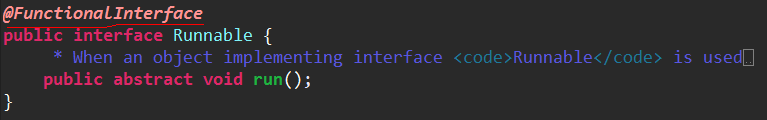b.用户自定义函数接口(注解必须有，表达式是直接通过参数列表来实现的，只能有一个有效方法)

``````
@FunctionalInterface
public interface MyInterface {
String info(String tip);
}``````

5.公共定义的函数式接口

a.功能性接口：Function<T, R>

``````package org.xxxx.demo01;

import java.util.function.Function;

public class Demo01 {
public static void main(String[] args) {
// 定义字符串
String str = "helloworld";

// 调用方法
// 在调用的时候写方法体，方法比较灵活
int length = testFun(str, (s) -> s.length());

System.out.println(length);
}

// 方法
/**
*
* @param str 输入参数
* @param fun 表达式 String 为输入类型，Integer为输出类型
* @return 返回字符串长度
*/
public static int testFun(String str, Function<String, Integer> fun) {
// 执行
Integer length = fun.apply(str);

return length;
}
}``````

b.消费型接口：Consumer<T>

Consumer 使用accept对参数执行行为

``````
package org.xxxx.demo01;

import java.util.function.Consumer;

public class Demo01 {
public static void main(String[] args) {
// 创建字符串
String str = "hello world";

// 调用
testCon(str, (s) -> System.out.println(s));
}

/**
*
* @param str 传入参数
* @param con
*/
public static void testCon(String str, Consumer<String> con) {
// 执行
con.accept(str);
}

}``````

c.供给型接口：Supplier<T>

``````package org.xxxx.demo01;

import java.util.function.Supplier;

public class Demo01 {
public static void main(String[] args) {
// 创建字符串
String str = "hello world";

// 调用
String sup = testSup(() -> str);

System.out.println(sup);
}

/**
*
* @param sup
* @return
*/
public static String testSup(Supplier<String> sup) {
// 执行
String s = sup.get();
return s;
}

}``````

d.断言型接口：Predicate<T>

``````package org.xxxx.demo01;

import java.util.function.Predicate;

public class Demo01 {
public static void main(String[] args) {
// 创建字符串
String str = "hello world";

// 调用
boolean flag = testPre(str, (s) -> s.length() > 0);

System.out.println(flag);
}

/**
*
* @param str
* @param pre
* @return
*/
public static boolean testPre(String str, Predicate<String> pre) {
// 执行
boolean flag = pre.test(str);

return flag;
}

}``````

6.Lambda的优点

a.极大的减少代码冗余，同时可读性也好过冗长的匿名内部类

b.与集合类批处理操作结合，实现内部迭代，并充分利用现代多核CPU进行并行计算。之前集合类的迭代都是外部的，即客户代码。而内部迭代意味着由Java类库来进行迭代，而不是客户代码

7.和匿名内部类的区别

a.在lambda中，this不是指向lambda表达式产生的那个对象，而是它的外部对象

b.Java 编译器编译 Lambda 表达式并将他们转化为类里面的私有函数，它使用 Java 7 中新加的 invokedynamic 指令动态绑定该方法，但每一个匿名内部类编译器会为其创建一个类文件

B.方法引用

1.概述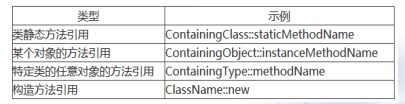forEach()也是jdk8的新特性

2.类静态方法引用

``````package org.xxxx.demo01;

import java.util.function.Function;

public class Demo01 {
public static void main(String[] args) {
// 调用
long l1 = testAbs(-10L, (s) -> Math.abs(s));

// 改进
long l2 = testAbs(-10, Math::abs);

System.out.println(l1);
System.out.println(l2);
}

public static long testAbs(long s, Function<Long, Long> fun) {
Long l = fun.apply(s);
return l;
}
}``````

3.实例方法引用

(s) -> System.out.println(s)的类型是Consumer类型

``````
package org.xxxx.demo01;

import java.util.ArrayList;
import java.util.List;

public class Demo01 {
public static void main(String[] args) {
// 创建集合
List<String> list = new ArrayList<>();

// 添加元素

// 排序
list.sort((s1, s2) -> s1.compareTo(s2));

// 遍历
list.forEach((s) -> System.out.println(s));
list.forEach(System.out::println);
}
}``````

4.构造方法引用

``````
package org.xxxx.demo01;

import java.util.function.Supplier;

public class Demo01 {
public static void main(String[] args) {
getString(String :: new);
}

public static void getString(Supplier<String> su) {
String s = su.get();
System.out.println(s == null);
}
}``````

5.任意对象的实例方法

C.Stream流

1.思考

a.代码冗余

b.实现并行计算很麻烦

c.代码无法及时传递程序员的意图 ，必须读完代码

2.外部迭代

forEach工作原理：代码底层使用Iterator进行迭代的，是串行化操作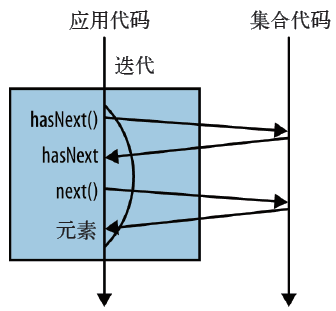3.内部迭代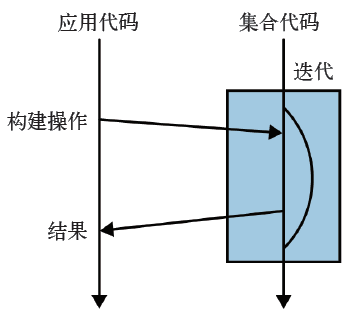4.Stream概述

Stream 会隐式地在内部进行遍历，做出相应的数据转换

5.常用操作

a.forEach

b.collect(toList())

``````package org.xxxx.demo01;

import java.util.ArrayList;
import java.util.List;
import java.util.stream.Collectors;

public class Demo01 {
public static void main(String[] args) {
// 创建集合
List<String> list = new ArrayList<>();

// 添加元素

// 统计长度大于2的
long count = list.stream().filter((s) -> s.length() > 2).count();

// 将符合要求的放入集合
List<String> list2 = list.stream().filter((s) -> s.length() > 2).collect(Collectors.toList());

System.out.println(count);
list2.forEach(System.out :: println);
}

}``````

6.map

map 操作就可以使用该函数，将一个流中的值转换成一个新的流

``````package org.xxxx.demo01;

import java.util.ArrayList;
import java.util.List;
import java.util.stream.Collectors;

public class Demo01 {
public static void main(String[] args) {
// 创建集合
List<String> list = new ArrayList<>();

// 添加元素

// 转换为大写
List<String> list2 = list.stream().map((s) -> s.toUpperCase()).collect(Collectors.toList());

list2.forEach(System.out :: println);

}

}
``````

7.filter

``````package org.xxxx.demo01;

import java.util.ArrayList;
import java.util.List;
import java.util.stream.Collectors;

public class Demo01 {
public static void main(String[] args) {
// 创建集合
List<String> list = new ArrayList<>();

// 添加元素

// 获取数字开头的
List<String> list2 = list.stream().filter((s) -> Character.isDigit(s.charAt(0))).collect(Collectors.toList());

list2.forEach(System.out::println);
}

}``````

8.flatMap

map 操作可用一个新的值代替Stream 中的值

``````package org.xxxx.demo01;

import java.util.Arrays;
import java.util.stream.Stream;

public class Demo01 {
public static void main(String[] args) {

Stream<?> flatMap = Stream.of(Arrays.asList("a", "b"), Arrays.asList(1, 2, 3)).flatMap((s) -> s.stream());
flatMap.forEach(System.out :: println);
}

}``````

9.max和min

``````package org.xxxx.demo01;

import java.util.ArrayList;
import java.util.Comparator;
import java.util.List;

public class Demo01 {
public static void main(String[] args) {
List<String> list = new ArrayList<>();

// 获取最大值
int max = list.stream().map((s) -> s.length()).max(Integer :: compareTo).get();
System.out.println(max);

// 获取最小值，另一种方法
int min = list.stream().min(Comparator.comparing((s) -> s.length())).get().length();
System.out.println(min);
}

}``````

10.reduce

``````package org.xxxx.demo01;

import java.util.ArrayList;
import java.util.List;

public class Demo01 {
public static void main(String[] args) {
List<Long> list = new ArrayList<>();

// 封装到集合
for (long i = 1; i <= 100; i++) {
}

// 计算
// reduce：参1，和的初始值
Long sum = list.stream().parallel().reduce(0L, (r, l) -> r + l);

System.out.println(sum);
}

``````

11.练习

a.获取Student集合中年龄小于20岁的集合中年龄最大的学生信息

Student类省略

``````package org.xxxx.demo01;

import java.util.ArrayList;
import java.util.Comparator;
import java.util.List;

public class Demo01 {
public static void main(String[] args) {
List<Student> list = new ArrayList<>();

Student s1 = new Student("张三", 21);
Student s2 = new Student("李四", 19);
Student s3 = new Student("王五", 18);
Student s4 = new Student("程六", 17);
Student s5 = new Student("赵七", 20);

// 筛选
Student student = list.stream().filter((s) -> s.getAge() < 20).max(Comparator.comparing((s) -> s.getAge()))
.get();
System.out.println(student);
}

}``````

b.查找集合中以a开头的字符的长度集合

``````package org.xxxx.demo01;

import java.util.List;
import java.util.stream.Collectors;
import java.util.stream.Stream;

public class Demo01 {
public static void main(String[] args) {
List<Integer> list = Stream.of("abc", "b", "a", "abcd").filter((s) -> s.startsWith("a")).map((s) -> s.length())
.collect(Collectors.toList());
System.out.println(list);
}

}``````

D.并行API

1.概述

a.将数据分成部分

b.给每个子部分分配一个子线程

c.等待所有的子线程全部结束

d.合并子线程

Stream有串行和并行两种，串行Stream上的操作是在一个线程中依次完成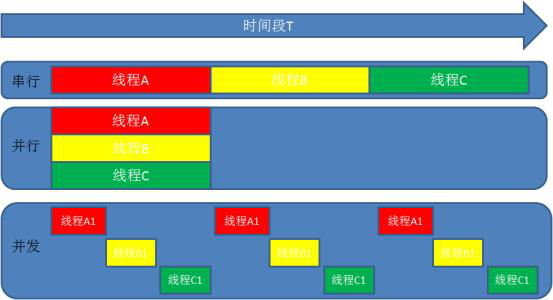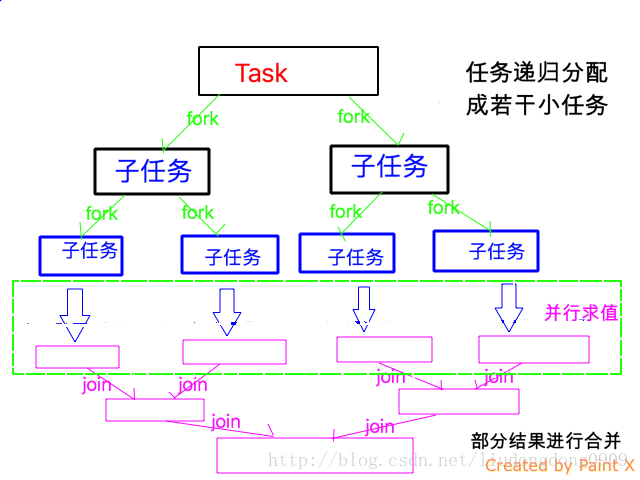2.使用方法

a.调用Stream的parallel()方法

b.调用Collection的parallelStream()方法

c.parallel() 与 sequential() 可在并行流与顺序流之间切换

``````package org.xxxx.demo01;

import java.util.ArrayList;
import java.util.List;

public class Demo01 {
public static void main(String[] args) {
List<Long> list = new ArrayList<>();

// 封装到集合
for (long i = 1; i <= 100; i++) {
}

// 计算
// reduce：参1，和的初始值
Long sum = list.stream().parallel().reduce(0L, (r, l) -> r + l);

System.out.println(sum);
}

}``````

3.使用并行的建议

a.尽量使用基本类型的流  IntStream, LongStream, and DoubleStream

b.有些操作使用并发流的性能会比顺序流的性能更差，比如limit，findFirst，依赖元素顺序的操作在并发流中是极其消耗性能的.findAny的性能就会好很多，因为不依赖顺序

c.考虑流中计算的性能(Q)和操作的性能(N)的对比， Q表示单个处理所需的时间， N表示需要处理的数量，如果Q的值越大, 使用并发流的性能就会越高

d.数据量不大时使用并发流，性能得不到提升

e.考虑数据结构：并发流需要对数据进行分解，不同的数据结构被分解的性能时不一样的

f.传递给并行流的函数都是线程安全的，无副作用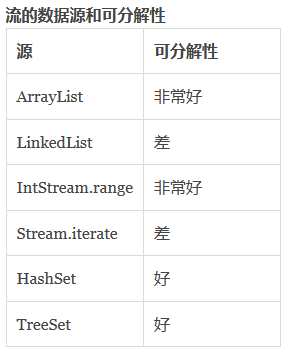4.何时需要并行编程

a.是否需要并行

b.任务之间是否是独立的？是否会引起任何竞态条件

c.结果是否取决于任务的调用顺序

E.Time日期时间

1.旧的日期时间缺点

a.非线程安全

java.util.Date不是线程安全的

b.设计不佳

c. 困难的时区处理

2.Instat

a.概述

b. Instant.now();获取当前时间

``````package org.xxxx.demo01;

import java.time.Instant;

public class Demo01 {
public static void main(String[] args) {
// 获取当前时间
Instant now = Instant.now();

// T代表东西经0°经线区时：伦敦时间
System.out.println(now);// 2017-11-25T14:06:57.079Z
}

}``````

c.Instant.ofEpochMilli(new Date().getTime()); 旧日期转为新日期

``````package org.xxxx.demo01;

import java.time.Instant;
import java.util.Date;

public class Demo01 {
public static void main(String[] args) {
// 获取当前时间
Instant instant = Instant.ofEpochMilli(new Date().getTime());
System.out.println(instant);
}

}``````

d. Instant.parse();日期文本格式转换为时间格式

``````package org.xxxx.demo01;

import java.time.Instant;

public class Demo01 {
public static void main(String[] args) {
// 获取当前时间
Instant instant = Instant.parse("1993-02-06T10:12:35Z");
System.out.println(instant);
}

}
``````

3.LocalDate

a.LocalDateTime.now();获取当前日期时间

b.now.minusDays(2);将当前日期时间减去两天

c.LocalDateTime.of(2016, 10, 23);构造一个指定日期时间的对象

``````package org.xxxx.demo01;

import java.time.LocalDate;

public class Demo01 {
public static void main(String[] args) {
// 当前时间
LocalDate now = LocalDate.now();
System.out.println(now);

// 往前推两天
LocalDate date = now.minusDays(2);
System.out.println(date);

// 制定一个日期
LocalDate localDate = LocalDate.of(1993, 2, 6);
System.out.println(localDate);
}

}``````

4.LocalTime

a. LocalTime.now();当前时间

b. LocalTime.of(22, 33);确定的时间

c.LocalTime.ofSecondOfDay(4503); 一天中的第4503秒

``````package org.xxxx.demo01;

import java.time.LocalTime;

public class Demo01 {
public static void main(String[] args) {
// 当前时间
LocalTime now = LocalTime.now();
System.out.println(now);

// 22:33
LocalTime localTime = LocalTime.of(22, 33);
System.out.println(localTime);

// 一天中的4503秒
LocalTime ofDay = LocalTime.ofSecondOfDay(4503);
System.out.println(ofDay);
}

}``````

5.LocalDateTim

是LocalDate和LocalTime的组合体，表示的是不带时区的日期及时间

a.LocalDateTime.now();当前时间

b.localDateTime.plusHours(25).plusMinutes(3); 当前时间加上25小时３分钟

c.LocalDateTime.of(2014, 1, 20, 3, 30, 20)；转换

``````package org.xxxx.demo01;

import java.time.LocalDateTime;

public class Demo01 {
public static void main(String[] args) {
// 当前时间
LocalDateTime now = LocalDateTime.now();
System.out.println(now);

// 当前时间加上25小时３分钟
LocalDateTime plusMinutes = now.plusHours(25).plusMinutes(3);
System.out.println(plusMinutes);

// 转换
LocalDateTime of = LocalDateTime.of(1993, 2, 6, 11, 23, 30);
System.out.println(of);
}

}``````

6.ZoneDateTime

a.ZonedDateTime.now();获取包含时区的当前日期时间

b.ZonedDateTime.of(LocalDateTime.of(2014, 1, 20, 3, 30, 20), ZoneId.of(“+08”));创建时区的日期时间对象

``````
package org.xxxx.demo01;

import java.time.LocalDateTime;
import java.time.ZoneId;
import java.time.ZonedDateTime;

public class Demo01 {
public static void main(String[] args) {
// 当前时间
ZonedDateTime now = ZonedDateTime.now();
System.out.println(now);

// 创建时区的日期时间对象
ZonedDateTime of = ZonedDateTime.of(LocalDateTime.of(1993, 2, 6, 11, 23, 30), ZoneId.of("+08"));
System.out.println(of);
}

}``````

7.DateTimeFormatter

a.格式化：日期对象转换为格式字符串

``````package org.xxxx.demo01;

import java.time.LocalDateTime;
import java.time.format.DateTimeFormatter;

public class Demo01 {
public static void main(String[] args) {
// 格式化
String time = DateTimeFormatter.ofPattern("yyyy.MM.dd HH:mm:ss").format(LocalDateTime.now());
System.out.println(time);
}

}``````

b.解析：格式字符串转换为日期对象

``````package org.xxxx.demo01;

import java.time.LocalDateTime;
import java.time.format.DateTimeFormatter;

public class Demo01 {
public static void main(String[] args) {
// 格式化
LocalDateTime parse = LocalDateTime.parse("2017.01.01 08:08:08", DateTimeFormatter.ofPattern("yyyy.MM.dd HH:mm:ss"));
System.out.println(parse);
}

}
``````

8. 遗留代码操作

a.Date –> Instant

b.Calendar–>Instant

``````package org.xxxx.demo01;

import java.time.Instant;
import java.util.Calendar;
import java.util.Date;

public class Demo01 {
public static void main(String[] args) {
Instant timestamp = new Date().toInstant();

Instant instant = Calendar.getInstance().toInstant();

System.out.println(timestamp);
System.out.println(instant);
}

}
``````

F.其他

1.默认方法

2.问：

a.选择父类方法。当父类提供具体实现时，接口同名同参方法被忽略

b.覆盖父类方法。当一个父接口提供默认方法，另一个父接口提供同名同参方法时候

3.静态方法

4.String新增join(字符串集合或数组)方法

``````package org.xxxx.demo01;

public class Demo01 {
public static void main(String[] args) {
String[] str = {"a", "b", "c"};

String join = String.join("-", str);
System.out.println(join);
}
}
``````

5.可能错过的Java7特性

try–with-resources

try-with-resources 能够很容易地关闭在 try-catch 语句块中使用的资源

try-with-resources 语句确保了每个资源在语句结束时关闭